# 13.2.3 Statistics (I), PT3 Focus Practice

Question 7:
Diagram below is an incomplete pictograph showing the sales of books for a duration of five months.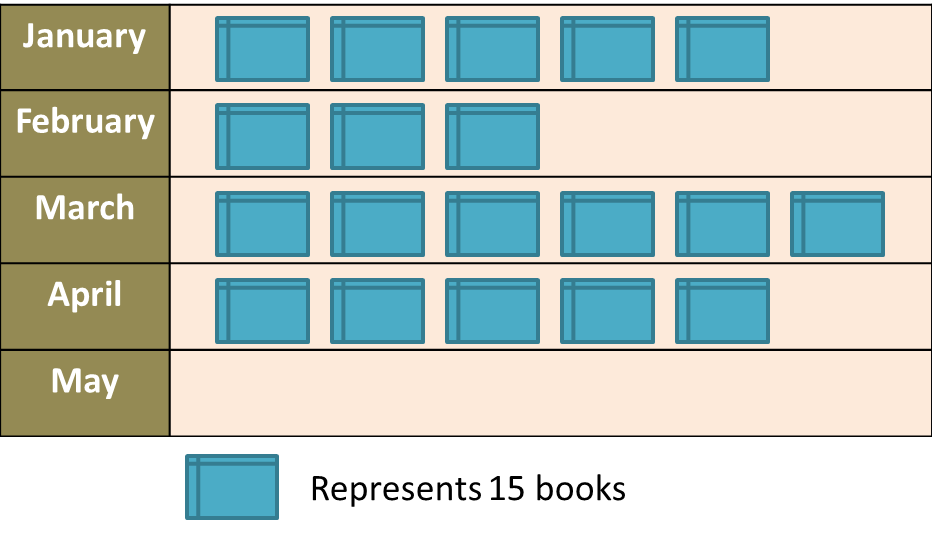(a) The sales in May is ¼ of the total sales in January and February.
Complete the pictograph in the Diagram.
(b) Find the total number of books sold before April.

Solution:
(a)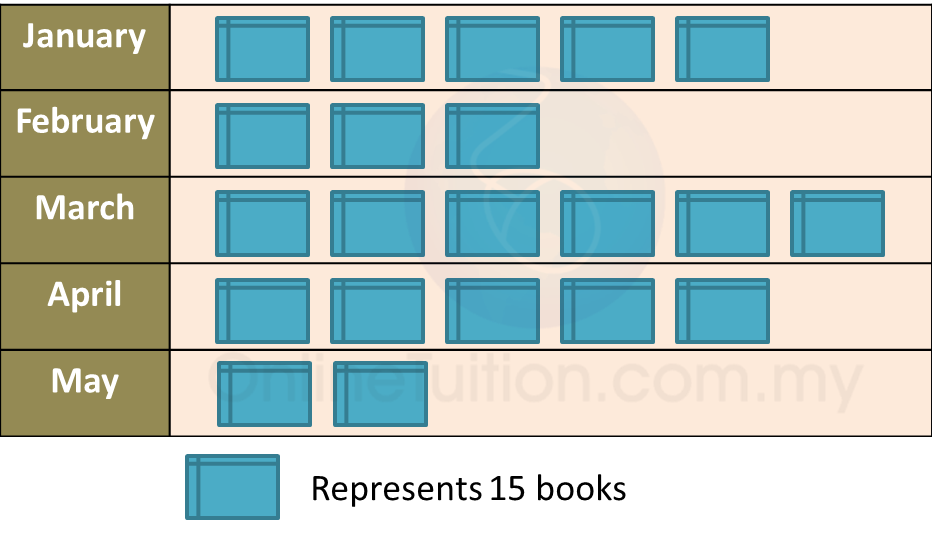(b)
Total number of books sold before April
= (5 + 3 + 6) × 15
= 14 × 15
= 210

Question 8:
Diagram below shows an incomplete line graph of the number of eggs sold in four weeks. The number of eggs sold on week 1 is 2000 and 4000 on week 4.(a) Complete the line graph in the Diagram.
(b) Complete the pie chart in the second Diagram to represent sales from Week 1 to Week 4.Solution:
(a)(b)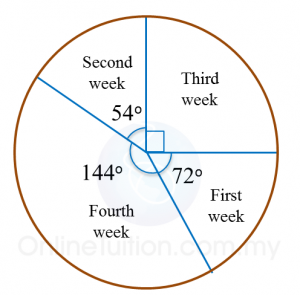# 13.2.2 Statistics (I), PT3 Focus Practice

 
 
13.2.2 Statistics (I), PT3 Focus Practice
 

 Question 4:
 
Table below shows the profit made from the sales of coconut at a stall over five days.
 

 
   Day     Day 1     Day 2     Day 3     Day 4     Day 5     Profit (RM)     280     200     200     360     320  
 
On diagram in the answer space, draw a line graph to represent all the information in the Table. Use the scale 2 cm to RM80 on the vertical axis.
 
 
: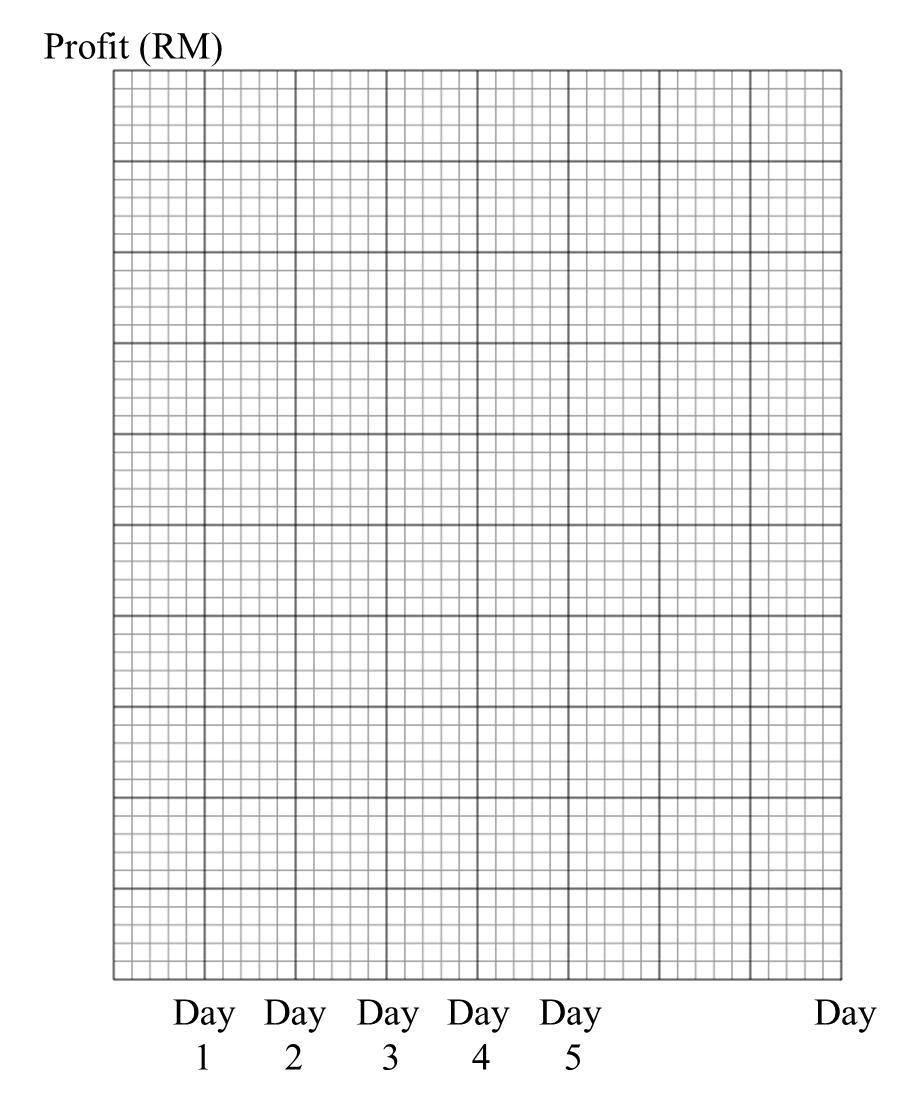Solution:Question 5:
 
Table below shows the number of students who read four types of books.
 
 
 
 
   Types of books     Fiction     Language     Technology     Religion     Number of students     18     16     8     14  
 
 
On diagram in the answer space, draw a bar chart to represent all the information in the Table.
 
 
: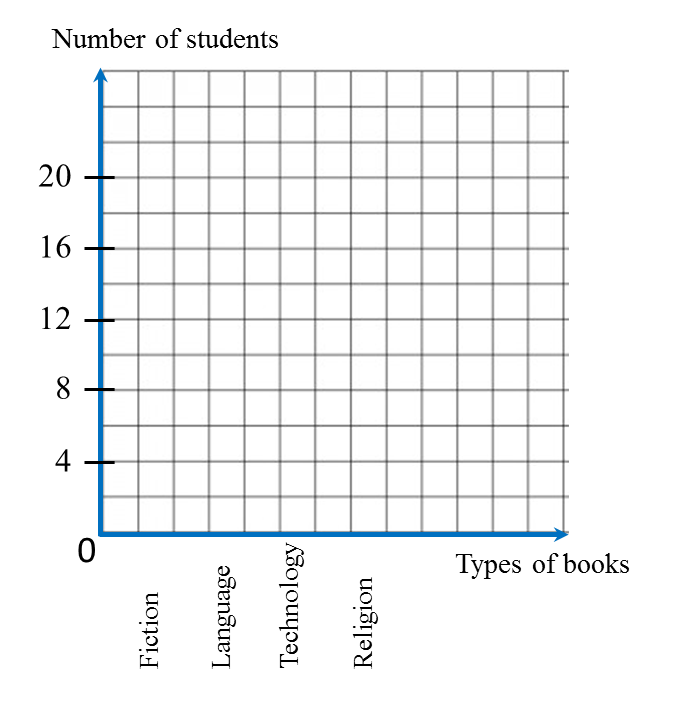Solution: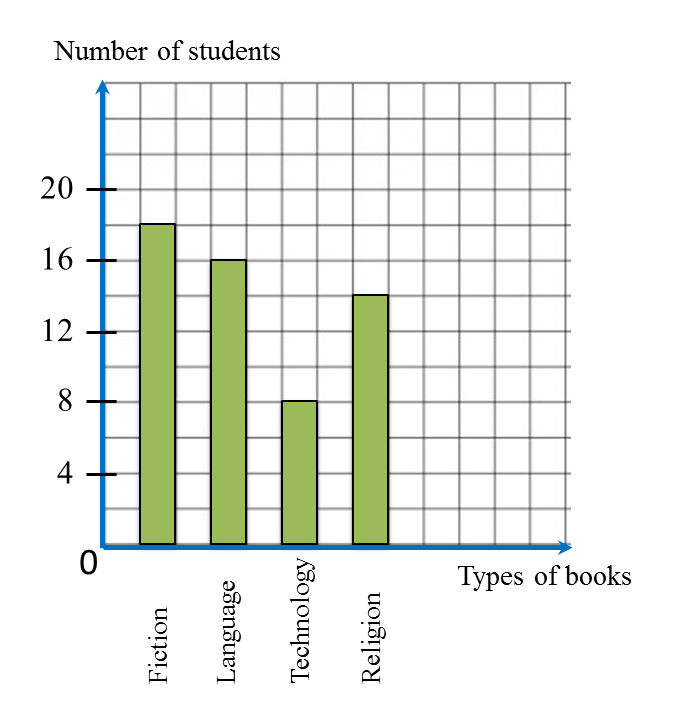Question 6:
Diagram below is an incomplete bar chart which shows the number of cars sold in a shop for duration of five months.(a) The number of cars sold for the five months is 150 units. The number of cars sold in February is equal to the number of cars sold in April.
Complete the bar chart in the Diagram.
(b) Find the percentage of cars sold in January.

Solution:
(a)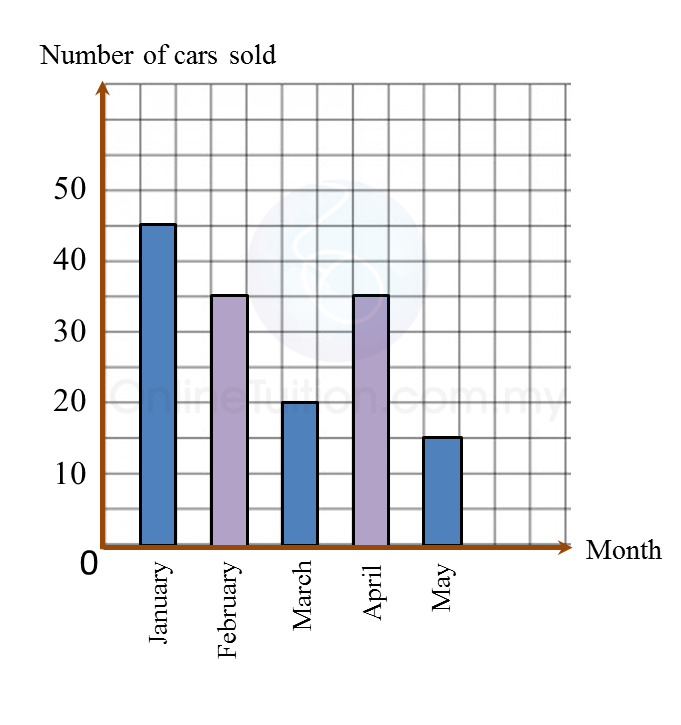(b)

# 4.7.6 Natural Polymers (Structured Questions)

Question 1:
Diagram 1.1 shows an experiment to study the effect of vinegar and ammonium solution on latex.
Diagram 1.2 shows the result of the experiment after 30 minutes.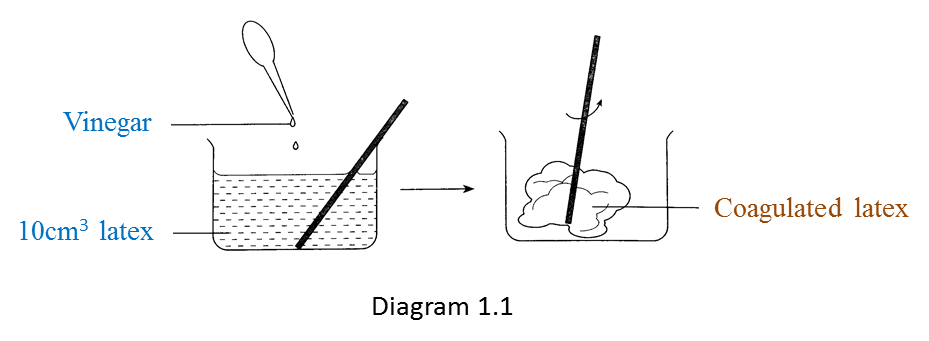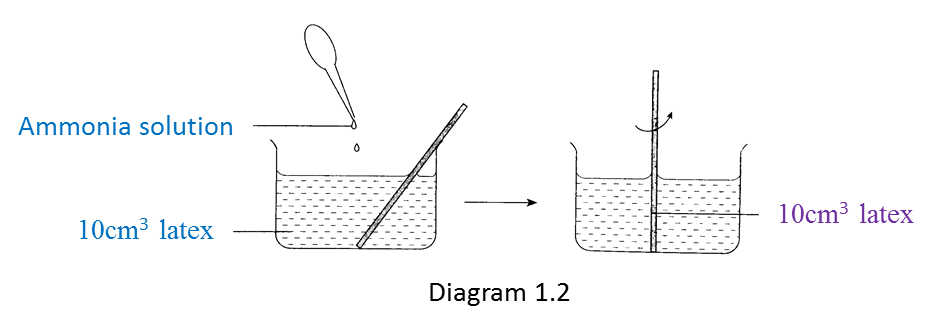(a) Write down one observation from the result of this experiment. [1 mark]

(b) State one inference based on the observation in Diagram 1.1. [1 mark]

(c) State one constant variable in this experiment. [1 mark]

(d) State one hypothesis for this experiment. [1 mark]

(e) Based on this experiment, state the operational definition for acid. [1 mark]

:
(a)
Vinegar causes the coagulation of latex but ammonia solution prevents the coagulation of latex.

(b)
When vinegar (an acid) is mixed with latex, the latex coagulates.
When ammonia solution (an alkali) is mixed with latex, the latex does not coagulate.
(any one)

(c)

1. 10 cm3 of latex
2. Results of the experiment are observed after 30 minutes
(any one)

(d)
Acid causes the coagulation of latex but alkali prevents the coagulation of latex.

(e)
Acid is a substance which causes latex to coagulate.

# 13.2.1 Statistics (I), PT3 Focus Practice

13.2.1 Statistics (I), PT3 Focus Practice
Question 1:
Diagram below is a pictograph showing the number of pizzas sold in three months.

The price of one pizza is RM18. The total sales for those four months are RM2250.
Calculate the number of pizzas sold in April.

Solution
:
Total number of pizzas sold in four months
$\begin{array}{l}=\frac{RM2250}{RM18}\\ =125\end{array}$

Total number of pizzas sold in first three months
= 9 × 10
= 90

Number of pizzas sold in April
= 125 – 90
= 35

Question 2:
Diagram below is a bar chart showing the number of new houses sold over four days.

The total number of new houses sold on Wednesday and Thursday is 56.
Calculate the total number of new houses sold on Monday and Tuesday.

Solution
:
Wednesday: 12 units
Thursday: 16 units
Number of houses represented by 1 unit
$\begin{array}{l}=\frac{56}{12+16}\\ =2\end{array}$
Monday: 14 units
Tuesday: 18 units

Total number of new houses sold on Monday and Tuesday
= (14 + 8) × 2
= 64

Question 3:
Table below shows the number of companies that gave contributions to an orphan fund over a period of five days.

 Days Monday Tuesday Wednesday Thursday Friday Number of companies 8 14 m 17 11

It is given that 20% of the total contribution was made on Tuesday.
Calculate the value of m

Solution
:
Let C = Total contribution
$\begin{array}{l}\frac{20}{100}×C=14\\ \text{}C=14×\frac{100}{20}\\ \text{}=70\\ m=70-\left(8+14+17+11\right)\\ \text{}=70-50\\ \text{}=20\end{array}$

# 4.7.4 The Oil Palm and Its Importance to National Development (Structured Questions)

Question 1:
Diagram 1.1 shows the process of latex coagulation.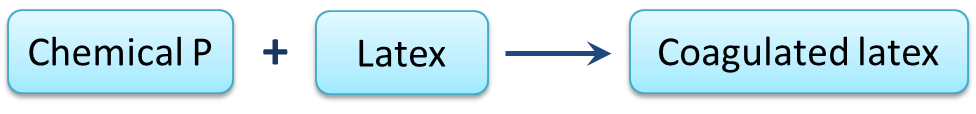(a)(i) State one example of chemical P? [1 mark]

(ii) State one characteristic of coagulated latex in Diagram 1.1. [1 mark]

(b) Chemical P in diagram 1.1 is replaced with chemical Q to prevent latex from coagulating.
State one example of chemical Q. [1 mark]

(c) Diagram 1.2 shows the process when natural rubber is heated with Sulphur to form rubber R.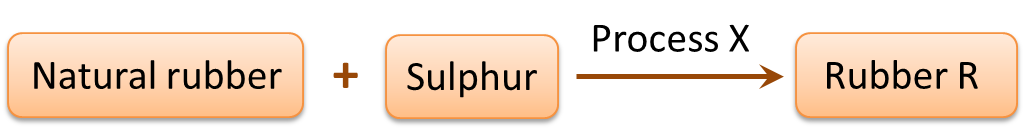(i) Name process X. [1 mark]
(ii) Name rubber R. [1 mark]

(d) Mark (\/) the object which is made of rubber R. [1 mark](a)(i)
Methanoic acid

(a)(ii)
Elastic

(b)
Ammonia solution

(c)(i)
Vulcanization of rubber

(c)(ii)
Vulcanized rubber

(d)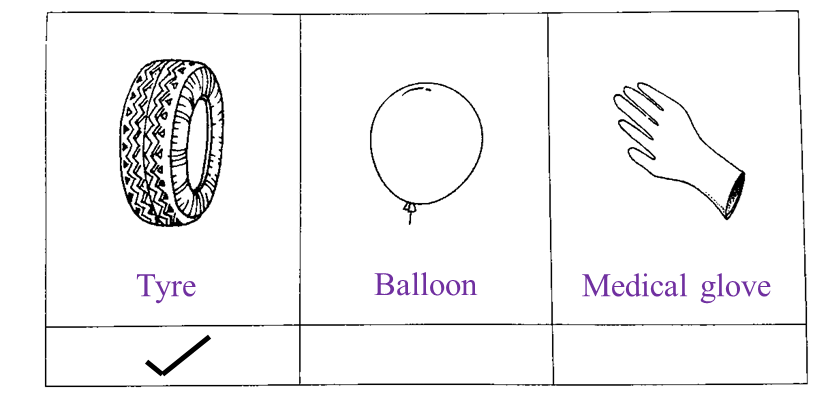# 4.7.2 Alcohol and Its Effects on Health (Structured Questions)

Question 1:
Diagram 1.1 and Diagram 1.2 show an experiment to investigate the effect of temperature on the fermentation of glucose by yeast.(a) State one hypothesis that can be made from this experiment. [1 mark]

(b) State the variables in this experiment.
(i) Manipulated variable [1 mark]
(ii) Responding variable [1 mark]

(c) Based on Diagram 1.1 and 1.2, which temperature is more suitable for the fermentation of glucose? [1 mark]

(d) Diagram 1.3 shows the graph of the volume of carbon dioxide produced at 35oC against time.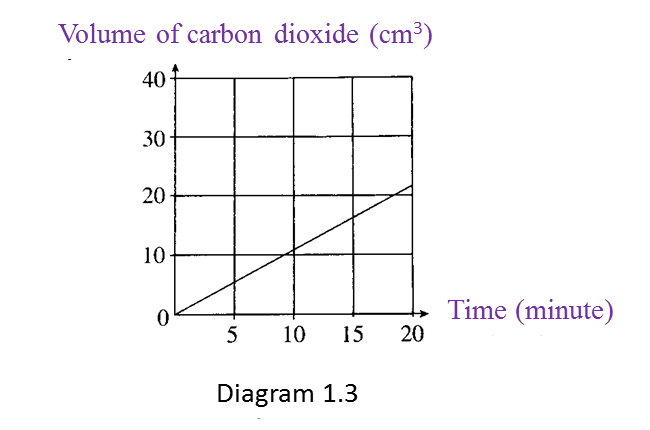What is the relationship between the volume of carbon dioxide produced and time? [1 mark]

(a) Fermentation of glucose by yeast is affected by temperature.

(b)(i)
Temperature of the water bath

(b)(ii)
Volume of carbon dioxide produced

(c)
35oC

(d)
The volume of carbon dioxide produced is directly proportional to time.

# 12.2.1 Solid Geometry (II), PT3 Focus Practice

12.2.1 Solid Geometry (II), PT3 Focus Practice

Question 1:
Diagram below shows closed right cylinder.

Calculate the total surface area, in cm2, of the cylinder.

$\left(\pi =\frac{22}{7}\right)$

Solution
:
Total surface area
= 2(πr2) + 2πrh
$\begin{array}{l}=\left(2×\frac{22}{7}×{7}^{2}\right)+\left(2×\frac{22}{7}×7×20\right)\\ =308+880\\ =1188c{m}^{2}\end{array}$

Question 2:
Diagram below shows a right prism with right-angled triangle ABC as its uniform cross section.
Calculate the total surface area, in cm2, of the prism.

Solution
:
$\begin{array}{l}AB=\sqrt{{5}^{2}-{3}^{2}}\\ =\sqrt{25-9}\\ =\sqrt{16}\\ =4cm\end{array}$

Total surface area
= 2 (½× 3 × 4) + (3 × 10) + (4 × 10) + (5 × 10)
= 12 + 30 + 40 + 50
= 132 cm2

Question 3:
Diagram below shows a right pyramid with a square base.

Calculate the total surface area, in cm2, of the right pyramid.

Solution
:
h2= 102 – 62
= 100 – 36
= 64
= √64
= 8cm

Total surface area of the right pyramid
= (12 × 12) + 4 × (½× 12 × 8)
= 144 + 192
= 336 cm2

Question 4:
The diagram shows a cone. The radius of its base is 3.5 cm and its slant height is 6 cm. Find the area of its curved surface.
( π= 22 7 )

Solution
:
Area of curved surface
= π × radius of base × slant height
= πrs
$\begin{array}{l}=\frac{22}{7}×3.5×6\\ =66c{m}^{2}\end{array}$

# 4.7.1 Various Carbon Compound (Structured Questions)

Question 1:
Diagram 1.1 shows two examples of carbon compounds, K and L.(a)(i) Based on Diagram 1.1, which one is an inorganic carbon compound? [1 mark]

(ii) State one characteristic of an inorganic carbon compound. [1 mark]

(b)(i) State one use of compound K. [1 mark]

(ii) State one effect of compound K on the nervous system if consumed excessively. [1 mark]

(c) Diagram 1.2 shows a tank containing gas M used for gas stoves.
Gas is a hydrocarbon compound.(i) State two elements present in gas M. [1 mark]

(ii) State one source of gas M. [1 mark]

(a)(i) L or marble chips

(a)(ii)
Originates from non-living things or does not originates from living things

(b)(i)
Alcoholic drink

(b)(ii)
Disrupts nerve coordination or slows down the transmission of impulses

(c)(i)
1. Hydrogen
2. Carbon

(c)(ii)
Petroleum